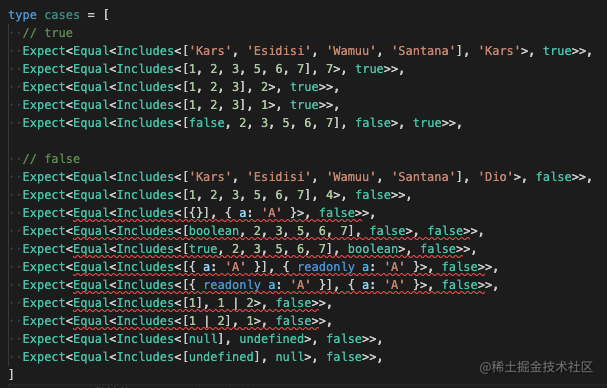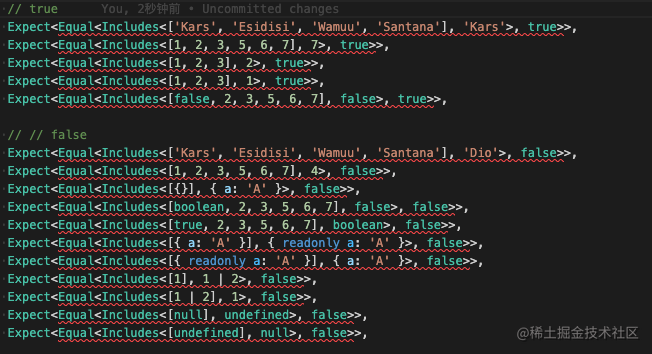# 精读TypeScript 类型体操练习 之 Includes

ts有时候遇到实际问题，看官方文档也是云里雾里的。基础能力很重要，学会了基础能力，但实际运用又不一定能想到这么用。解决问题最好的办法就是多思考多练，通过实际案例不断刺激大脑，养成ts思维习惯。推荐`github`上类型体操type challenges，空余时间有事没事练一两道题。

## Includes

#### 在js中

includes可以判断一个数组中是否包含某一个元素，并返回true或false

``````['a', 'b', 'c'].includes('a') // true

['a', 'b', 'c'].includes(1) // false

includes可以判断一个字符串中是否包含指定的子字符串，并返回true或false

``````const str = "Hello world"
str.includes('world') // true

#### 回到ts中

type Includes<T extends readonly any[], U> = any

``````type Includes<T extends readonly any[], U> = T extends U ? true : false

#### T extends U 为什么不成立？

T由于是数组类型，extends不支持判定`数组包含`逻辑。

#### 循环一个一个出来对比

``````type Includes<T extends readonly any[], U> = U extends T[number] ? true : false``````Expect<Equal<Includes<[boolean, 2, 3, 5, 6, 7], false>, false>>,
Expect<Equal<Includes<[true, 2, 3, 5, 6, 7], boolean>, false>>,

false exetnds boolean // false 继承了 boolean, 所以是true

boolean extends true // boolean 有可能是true也有可能是false，所以返回的依旧是boolean

``````Expect<Equal<Includes<[{}], { a: 'A' }>, false>>,

{ a: 'A' } extends {}

``````Expect<Equal<Includes<[{ a: 'A' }], { readonly a: 'A' }>, false>>,
Expect<Equal<Includes<[{ readonly a: 'A' }], { a: 'A' }>, false>>,

{ readonly a: 'A' } extends { a: 'A' } // { readonly a: 'A' }继承了{ a: 'A' }，所以是true

{ a: 'A' } extends { readonly a: 'A' } // 反过来{ a: 'A' }继承了{ readonly a: 'A' }，所以也是true

``````Expect<Equal<Includes<, 1 | 2>, false>>,
Expect<Equal<Includes<[1 | 2], 1>, false>>,

1 | 2 extends 1 // 1 既可以说是继承了 1 | 2, 也可以说不是，这个得看是1 还是2，所以返回的既是true也是false，也就是boolean

1 extends 1 | 2 // 1 | 2 肯定是继承了 1, 返回true``````type Includes<T extends readonly any[], U> = any

||

type Includes<T extends readonly any[], U> =
T extends [infer First, ...infer Rest]
?
true
:
false

``````Equal

``````type Includes<T extends readonly any[], U> =
T extends [infer First, ...infer Rest]
?
true
:
false

||

type Includes<T extends readonly any[], U> =
T extends [infer First, ...infer Rest]
?
Equal<First, U> extends true
? true
: Includes<Rest, U>
:
false
// 如果First也就是第一个就是true那么整体就是true，如果不成立那就递归，把剩下的递归给判断掉

``````Equal<X, Y> = any

``````如果 X extends Y 那就是true，不是就是不相等也就是false

``````Equal<X, Y> = X extends Y ? true : false

Equal类型这样就结束了吗？等等，还没有。

``````Expect<Equal<Includes<[true, 2, 3, 5, 6, 7], boolean>, false>>,
Expect<Equal<Includes<[{ a: 'A' }], { readonly a: 'A' }>, false>>,
Expect<Equal<Includes<[{ readonly a: 'A' }], { a: 'A' }>, false>>,
Expect<Equal<Includes<, 1 | 2>, false>>,

``````type Equal<X, Y> =
() extends () ? true : false

||

() // 括号里的写法

(<Res>() => Res extends X ? true : false) // 这样的写法也就是说用固定的true 或者 false 去跟 true 或者 false去对比是不是继承关系。

type Equal<X, Y> =
(<Res>() => Res extends X ? true : false)
extends
(<Res>() => Res extends Y ? true : false)
?
true
:
false

#### 最终答案

``````type Equal<X, Y> = (<Res>() => Res extends X ? ture : false) extends (<Res>() => Res extends Y ? true : false) ? true : false

type Includes<T extends readonly any[], U> =
T extends [infer First, ...infer Rest]
?
(
Equal<First, U> extends true
? true
: Includes<Rest, U>
)
:
false